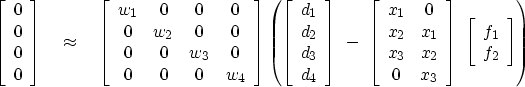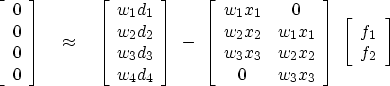Next: Meet the Toeplitz matrix Up: WEIGHTED ERROR FILTERS Previous: Automatic gain control

## Gain before or after convolution

It is a common but questionable practice to apply AGC to echo soundings before filter analysis. A better practice is first to analyze according to the laws of physics and only at the last stage to apply gain functions for purposes of statistical estimation and final display. Here we will examine correct and approximate ways of setting up deconvolution problems with gain functions. Then we will use CG to solve the proper formulation.

Solving problems in the time domain offers an advantage over the frequency domain because in the time domain it is easy to control the interval where the solution should exist. Another advantage of the time domain arises when weighting functions are appropriate. I have noticed that people sometimes use Fourier solutions inappropriately, forcing themselves to use uniform weighting when another weighting would work better. Since we look at echos, it is unavoidable that we apply gain functions. Weighting is always justified on the process outputs, but it is an approximation of unknown validity on the data that is input to those processes. I will clarify this approximation by an equation with two filter points and an output of four time points. In real-life applications, the output is typically 1000-2000 points and the filter 5-50 points. The valid formulation of a filtering problem is(18)
The weights wt are any positive numbers we choose. Typically the wt are chosen so that the residual components are about equal in magnitude.

If, instead, the weighting function is applied to the inputs, we have an approximation that is somewhat different:(19)
Comparing the weighted output-residual equation (18) to the weighted input-data equation (19), we note that their right-hand columns do not match. The right-hand column in (18) is (0, w2 x1, w3 x2, w4 x3)' but in (19) is (0, w1 x1, w2 x2, w3 x3)'. The matrix in (19) is a simple convolution, so some fast solution methods are applicable.Next: Meet the Toeplitz matrix Up: WEIGHTED ERROR FILTERS Previous: Automatic gain control
Stanford Exploration Project
10/21/1998